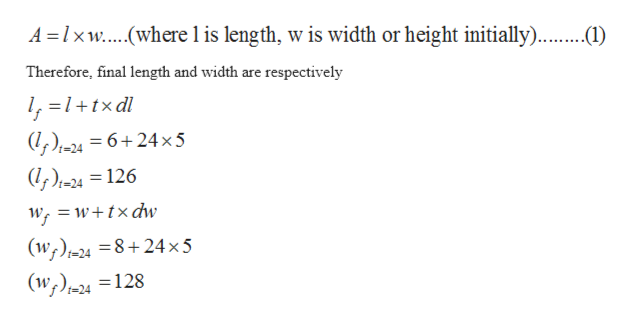# A rectangle initially has dimensions 6cm by 8 cm. All sides begin increasing in length at a rate of 5 cm/s. At what rate is the area of the rectangle increasing after 24 s​?Let​ A, b, and h be the​ area, base, and height of a​ rectangle, respectively. Write an equation relating​ A, b, and h.

Question
138 views
A rectangle initially has dimensions 6cm by 8 cm. All sides begin increasing in length at a rate of 5 cm/s. At what rate is the area of the rectangle increasing after 24 s​?
Let​ A, b, and h be the​ area, base, and height of a​ rectangle, respectively. Write an equation relating​ A, b, and h.
check_circle

Step 1

The initial dimensions of rectangle are 6cm by 8cm, where length is 6cm and width is 8cm.

Step 2

To determine at what rate the area of rectangle is increasing after 24 seconds.

Step 3

The area of rectangl...help_outlineImage TranscriptioncloseA lxw...(where l is length, w is width or height initially). Therefore, final length and width are respectively /, =1+tx dl (l,)-24 = 6+24x5 |(l, )1=24 = 126 W w+txdw (w)24 =8+24 x 5 (w)24 = 128 fullscreen

### Want to see the full answer?

See Solution

#### Want to see this answer and more?

Solutions are written by subject experts who are available 24/7. Questions are typically answered within 1 hour.*

See Solution
*Response times may vary by subject and question.
Tagged in

### Derivative